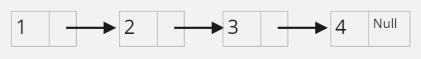# Linked List in C : Implementation

Linked List is a Linear Data Structure, Just Like the Array but in Array, Elements are stored in the Contiguous memory Location whereas in Linked List they are not stored in this manner.

In the linked list the elements are linked with each other using pointers. It consists of items called “Nodes” which contain two parts. The first part stores the actual data and the second part has a pointer that points to the next node.

The structure of a linked list is shown in the below image.

Every element in the Linked List is called a Node. A Node has a value associated with it and the pointer pointing to the next Node. Let us define a Node using struct which has an int and Node* as its data members.

This is how we define a node in C using structures or “struct”.

```struct Node
{
int val;
struct Node *next;
};```
```Linked List with 4 Nodes. ```

Following operations are performed on Linked List.

## C Program

``````void InsertatLast(int x) {

struct Node * temp;
temp = (struct Node * ) malloc(sizeof(struct Node));
temp -> val = x;
temp -> next = NULL;
tail = temp;
} else {
tail -> next = temp;
tail = tail -> next;
}
}``````

Time Complexity: O(N)

Space Complexity: O(1)

Code:

## C Program

``````void InsertatFirst(int x) {
struct Node * temp = (struct Node * ) malloc(sizeof(struct Node));
temp -> val = x;
temp -> next = NULL;
tail = temp;
} else {
}
}``````

Time Complexity: O(1)

Space Complexity: O(1)

Code:

## C Program

``````void InsertAfter(int pos, int x) {
struct Node * temp = (struct Node * ) malloc(sizeof(struct Node));
temp -> val = x;
temp -> next = NULL;
int len = Length();
if (pos > len) {
printf("Pos is Invalid\n");
printf("Linked List is having only %d Nodes \n", len);
return;
}
struct Node * curr = head;
while (--pos)
curr = curr -> next;
temp -> next = curr -> next;
curr -> next = temp;
}``````

Time Complexity: O(N)

Space Complexity: O(1)

Code:

## C Program

``````void Delete(int pos) {
int len = Length();//it gives the length of the linked list
if (pos > len) {
printf("Position is Invalid\n");
printf("Linked List is having only %d Nodes \n", len);
return;
} else if (pos == 1) {
struct Node * temp = head;
temp -> next = NULL;
free(temp);
} else {
struct Node * curr = head, * prev = NULL;
int loc = pos;
while (--loc) {
prev = curr;
curr = curr -> next;
}
prev -> next = curr -> next;
curr -> next = NULL;
free(curr);
if (pos == len)
tail = prev;
}
}``````

Time Complexity: O(N)

Space Complexity: O(1)

Code:

## C Program

``````void Traverse() {
struct Node * curr = head;
printf("[ ");
if (curr == NULL) {
return;
}
while (curr != NULL) {
printf("%d ", curr -> val);
curr = curr -> next;
}
printf(" ]");
printf("\n");
}``````

Time Complexity: O(N)

Space Complexity: O(1)

Code:

## C Program

``````int Length() {
int nodescount = 0;
struct Node * curr = head;
for (; curr != NULL; curr = curr -> next)
nodescount++;
return nodescount;
}``````

Time Complexity: O(N)

Space Complexity: O(1)

Code:

## C Program

``````void ReverseList() {
struct Node * third = NULL, * second = NULL, * first = head;
while (first) {
third = second;
second = first;
first = first -> next;
second -> next = third;
}
}``````

Time Complexity: O(N)

Space Complexity: O(1)

Code:

## C Program

``````struct Node * SearchForValue(int x) {
struct Node * curr = head;
while (curr) {
if (curr -> val == x)
return curr;
curr = curr -> next;
}
return NULL;
}``````

Time Complexity: O(N)

Space Complexity: O(1)